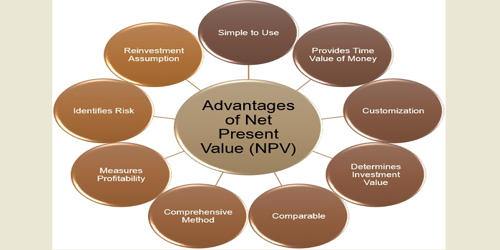Accounting

# Advantages of Net Present Value (NPV)Net Present Value (NPV) is one of the discounted cash flow techniques used in capital budgeting to determine the viability of a project or an investment. NPV lets you know whether the value of all cash flows that a project generates will exceed the cost of starting that particular project. NPV is the difference between the present value of cash inflows and the present value of cash outflows over a period of time.

A positive NPV denotes a good return and a negative NPV denotes a poor return. This process provides a straightforward way to analyze the profitability of a potential project of investment.

Advantages of Net Present Value (NPV)

(i) NPV gives importance to the time value of money. The primary advantage of using NPV is that it considers the concept of the time value of money i.e a dollar today is worth more than a dollar tomorrow owing to its earning capacity.

(ii) NPV method enables the decision-making process for companies. Not only does it help evaluate projects of the same size, but it also helps in identifying whether a particular investment is profit-making or loss-making.

(iii) In the calculation of NPV, both after cash flow and before cash flow over the life span of the project are considered. The entire computation of NPV rests on discounting the future cash flows to its present value using the required rate of return.

(iv) Profitability and risk of the projects are given high priority. It considers risk and capital costs when making future projections. This advantage is the reason behind the unique accuracy that the NPV holds as an evaluation tool for agencies and investors.

(v) NPV helps in maximizing the firm’s value. Net Present Value is a critical component of what is called the “profitability index.” If you wish to choose one single project from amongst many then NPV will be a good measure of profitability.

(vi) NPV takes into account each and every cash flow you define. It’s not like the payback period method or discounted payback period method which ignores cash flows beyond the payback period. This advantage means that investors have access to valuable data to help them determine if a specific activity makes sense.

(vii) Investments frequently occur in the corporate world. The Net Present Value does more than calculate an equity investment for individuals. Businesses can also use this ratio to determine if specific projects are worth a future investment.

(viii) Using Net Present Value makes sense for investors because it doesn’t assume that cash flows will automatically go into the Internal Rate of Return (IRR). IRR is the interest rate at which the NPV of all cash flows, both positive and negative, equal zero.

Discount rates are used in calculating NPV; the risk of undertaking the project (Business risk, financial risk, operating risk) gets factored into this method. It is the difference between the total present value of future cash inflows and the total present value of future cash outflows. Thus, it calculates the present value of future cash flows in excess of the present value of the investment outlay.